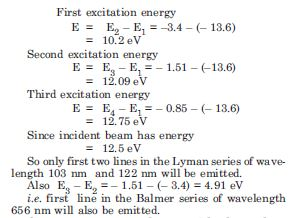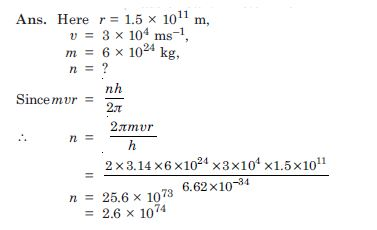# NCERT Solutions for Class 12 Physics Chapter 2 - Atoms

The NCERT Solutions at Aasoka are beneficial for students of Class 12 to learn and understand the concept better. They can enhance their overall performance to achieve good scores in the board exams. Students can refer to NCERT Solutions for Class 12 to get started with their learning journey and revise the concepts included in the board exams. Along with the board exams, they can also prepare for competitive exams including NEET and JEE.

“Atoms” chapter of Physics Class 12 includes important formulas which students need to memorize to achieve good scores in their board exams. The chapter consists of topics like Rutherford’s model of atom, hydrogen spectrum, Bohr model, Alpha-particle scattering experiment, energy levels, etc. In this chapter, students must stay special attention to the Bohr Model of Hydrogen Atom as it is an important topic for the board exam.

##### Question 1:

Choose the correct alternative from the clues given at the end of each statement :

1. The size of the atom in Thomson’s model is ..............the atomic size in Rutherford’s model.
(much greater than, not different from, much less than).
2. In the ground state of............... electrons are in the stable equilibrium, while in...........electrons always experience a net force
(Thomson’s model, Rutherford’s model).
3. A classical atom based on..........is doomed to collapse.
(Thomson’s model, Rutherford’s model)
4. An atom has nearly continuous mass distribution in a........... but has a highly non-uniform mass distribution in.......
(Thomson’s model, Rutherford’s model).
5. The positively charge part of the atom possesses most of the mass in.........
(Rutherford’s model, both the models).

(a) Not different from (b)Thomson’s model. Rutherford model (c) Rutherford’s model (d) Thomson’s model, Rutherford model (e) both the models.

##### Question 2:

Suppose you are given a chance to repeat the alpha-particle scattering experiment using a thin sheet of solid hydrogen in place of the gold foil. (Hydrogen is a solid at temperatures below 14 K). What result do you expect ?

The nucleus of hydrogen atom is a proton.
The mass of proton is 1.67 × 10–27 kg, whereas the mass of an incident α–particle is 6.64 × 10–27 kg. Because the scattering part is more massive than the target nuclei (proton), the α–particle won’t bounce back in even head-on collision. It is similar to a football colliding with a tennis ball at rest. Thus there would be no large angle scattering.

##### Question 3:

What is the shortest wavelength present in the Paschen series of spectral lines ?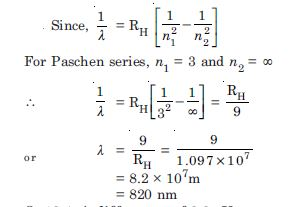##### Question 4:

A difference of 2.3 eV separates two energy levels in an atom. What is the frequsency of radiation emitted when the atom makes a transition from the upper level to the lower level ?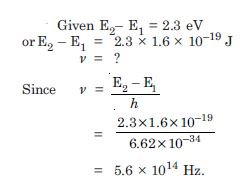##### Question 5:

The ground state energy of hydrogen atom is –13.6 eV. What are the kinetic and potential energies of the electron in this state ?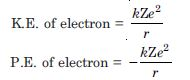##### Question 6:

A hydrogen atom initially in the ground level absorbs a photon, which excites it to the n = 4 level. Determine the wavelength and frequency of photon.

The energy of electron in nth state of hydrogen atom is given by :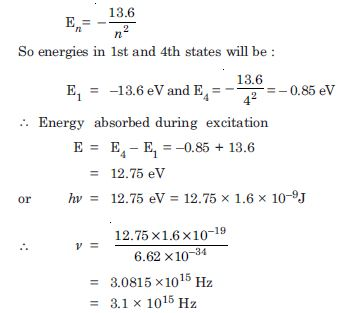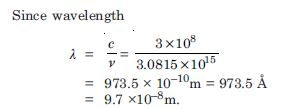##### Question 7:

Using the Bohr’s model, calculate the speed of the electron in a hydrogen atom in the n = 1, 2 and 3 levels Calculate orbital period in each of these levels.

The velocity of electron in nth orbit in an atom is given by :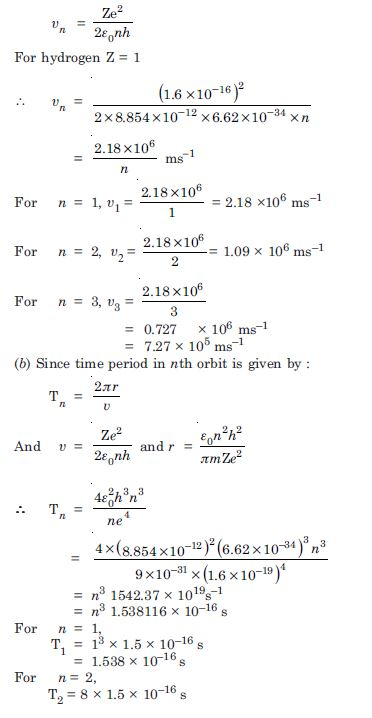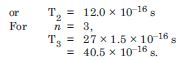##### Question 8:

The radius of the innermost electron orbit of a hydrogen atom is 5.3 ×10–11m. What are the radii of the n = 2 and n = 3 orbits ?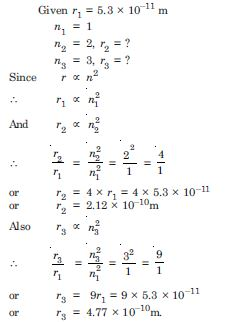##### Question 9:

A 12.5 eV electron beam is used to bombard gaseous hydrogen at room temperature. What series wavelength will be emitted ?